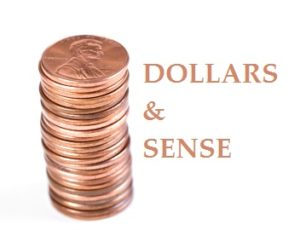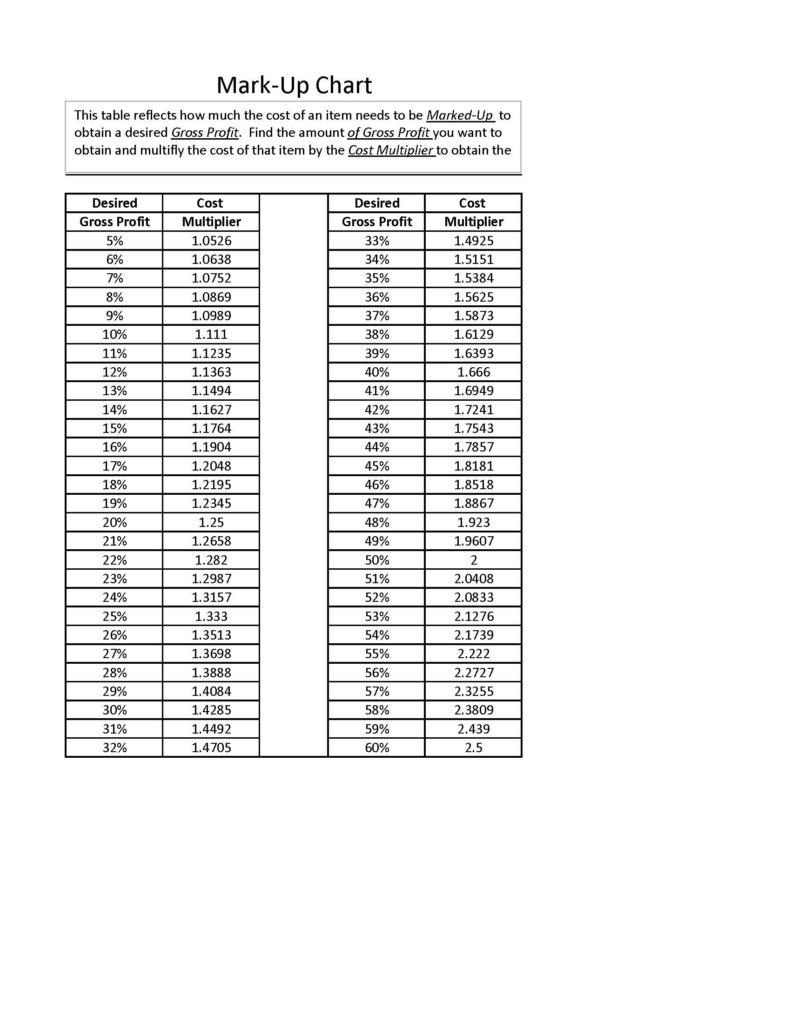##Margins and markup can lead to considerable confusion when setting product and service prices.  Margins and markups are terms used to determine cost and profits.  Not using these two terms in a proper way can lead to setting prices that are too high or too low, resulting in lost revenue or lost profits.

Explanation of Margin and Markup are as follows:

** Margin (also known as gross margin) is sales minus the cost of goods sold.

Sale price – Cost = Margin

If an item sales for \$100.00 and the cost is \$70.00. the margin would be \$30.00.  To state as a percentage, the margin percentage would be 30% (calculated as the margin divided by sales).

Margin / Sales= Margin Percentage

** Markup is the dollar amount by which the cost of a product is increased in order to establish the selling price.  Using the above example a markup of \$30.00 from the \$70.00 cost gives us \$100.00 selling price.

Markup + Cost = Selling Price

The markup percentage being 42.9% (calculated as the markup amount, divided by the product cost.)

Markup / Cost = Markup Percentage

As you can see both methods of calculating margin and markup take into account the cost of the item and its selling price.  Margin and markup have different uses.  Usually a markup formula is used to set the price of a product.  Margin is used more for analyzing sales and expenses.  You would use data from a given period (such as the previous quarter) to determine how much margin is required for existing sales volume to cover added expenses plus an adequate profit.  The goal is to use margin analysis to adjust prices and determine what your markup formula should be.

### When Considering Cost

The cost of a product is a common factor for both margin and markup.  It consists of the purchase price of the item (raw materials) plus labor to manufacture.  Cost does not include other expenditures such as rent, administrative salaries, freight and office expenses.  To cover these expenses, a margin analysis must be done.  A margin analysis takes actual data on costs, sales and expenses and creates a model that allocates the proportion of expenses that the margin must cover.  Once this analysis has been done, you can effectively set or adjust the prices and create a useful markup formula for pricing.

### Considerations

Determining the right markup to set the price of a product is vital.  You do not want to under price or over price your products.  Keeping track of margins on a regular basis enables you to avoid both problems.  Using margins to analyze sales and expenses can also help you to identify products that are not “pulling their weight”.  This will also enable you to establish how much you can offer in discounts and other promotional items to build customer bases and sales volume without discounting too deeply.